# The Gyroelongated Pentagonal Birotunda

The gyroelongated pentagonal birotunda is the 48th Johnson solid (J48). It has 40 vertices, 90 edges, and 52 faces (40 equilateral triangles and 12 pentagons).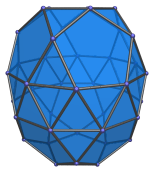The gyroelongated pentagonal birotunda can be constructed by attaching two pentagonal rotundae to a decagonal antiprism, or equivalently, adding a pentagonal rotunda to a gyroelongated pentagonal rotunda (J25).

J48 is one of the few Johnson solids that are chiral; it is distinct from its own mirror image: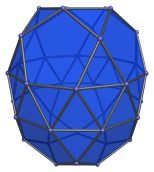## Projections

Here are some views of the gyroelongated pentagonal birotunda from various angles:

Projection Description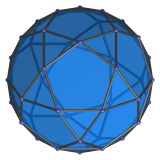Top view.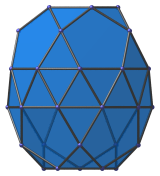Side view.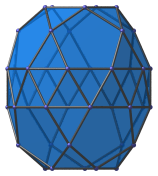9° side view.

## Coordinates

The Cartesian coordinates of the gyroelongated pentagonal birotunda with edge length 2 are:

• (0, −√((10+2√5)/5), H+√((20+8√5)/5))
• (±1, √((5+2√5)/5), H+√((20+8√5)/5))
• (±φ, −√((5−√5)/10), H+√((20+8√5)/5))
• (0, √((20+8√5)/5), H+√((10+2√5)/5))
• (±φ, −√((25+11√5)/10), H+√((10+2√5)/5))
• (±φ2, √((5+√5)/10), H+√((10+2√5)/5))
• (±1, ±√(3+4φ), H)
• (±φ2, ±√(2+φ), H)
• (±2φ, 0, H)
• (0, ±2φ, −H)
• (±√(3+4φ), ±1, −H)
• (±√(2+φ), ±φ2, −H)
• (√((20+8√5)/5), 0, −(H+√((10+2√5)/5)))
• (−√((25+11√5)/10), ±φ, −(H+√((10+2√5)/5)))
• (√((5+√5)/10), ±φ2, −(H+√((10+2√5)/5)))
• (−√((10+2√5)/5), 0, −(H+√((20+8√5)/5)))
• (√((5+2√5)/5), ±1, −(H+√((20+8√5)/5)))
• (−√((5−√5)/10), ±φ, −(H+√((20+8√5)/5)))

where H = √(√(11φ+7)−2φ−1), approximately 0.862397, is half the height of a decagonal antiprism of edge length 2, and φ = (1+√5)/2 is the Golden Ratio.

Last updated 19 Jun 2018.